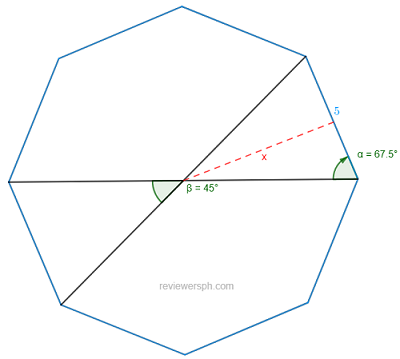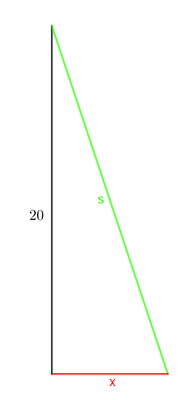### Math Notes

Subjects

#### Plane Geometry Solutions

##### Topics || Problems

A pyramid having a base of 5 cm and a perpendicular height of 20 cm. What is the total surface area?
Solution

The total surface area($$T_s$$) is the sum of the area of the base and the sum of the area of all the triangle sides.

$$T_s = A_b + A_s$$

Where: $$A_b$$ is the area of the base and $$A_s$$ is the area of the sides

Calculate the area of the base:To calculate the central angle: $$\frac{360}{8} = 45$$

To calculate the base angles: $$\frac{180-45}{2} = 67.5$$

$$A_b =\frac{1}{2} (5^2)(\frac{\sin{67.5} \sin{67.5}}{\sin{45}}) (8)$$

Since there are 8 equal sides of an octagon, multiply the area of one triangle to 8.

$$A_b =120.71$$

Calculate the area of the sides:Since there are 8 sides the area of the side is $$8(\frac{bs}{2})$$, where $$b$$ is the lenght of the base and $$s$$ is the altitude of the triangle on the side.

Calculate $$s$$:

$$s^2 = x^2 + 20^2$$

$$\tan{67.5} = \frac{x}{2.5}$$

$$x = 2.5\tan{67.5}$$

$$s =20.89$$

$$A_s = 8\frac{1}{2} (5) (20.89)$$

$$A_s = 417.82$$

$$A_t = 120.71 + 417.82 = 538.53 ~cm^2$$